Mobile QR Code1. (Department of Electrical and Computer Engineering, Seoul National University, Seoul 08826, Korea)
2. (Ayar Labs Incoporated, CA 95054, US)
3. (Cadence Design Systems, CA, 95134, US)

Receiver front-end, inverter-based Cherry-Hooper amplifier with an inductor, avalanche photodiode

## I. INTRODUCTION

The requirement of increased data rate in the data center accelerates the development of IC chips targeting IEEE standards 100GbE specifications which are categorized by the length of the channels. Phenomena of skin effects and dielectric loss of the copper-based wires make long-distance and high-speed communication difficult. Therefore, an equalization process is required to compensate for this channel loss. On the other hand, the optical fiber offers low attenuation loss than the copper channel.

Although optical fiber has the advantage of low attenuation, there is additional loss called absorption loss. The loss by absorption increases in proportion to the data rate and the length of transmission. As a solution for this, improving optical sensitivity using avalanche photodiode (APD) instead of the photodiode (PD) has been studied . The APD generates a higher reverse diode current than the conventional PD by impact ionization. The mechanism of the APD is illustrated in Fig. 1(a). With the high reverse bias voltage in the APD, the diode enters close to the breakdown region, and the photocurrent of the APD increases rapidly by impact ionization. Fig. 1(b) shows the diode current according to the reverse bias voltage applied to the APD. In addition, the APD responsivity, R$_{responsivity}$, is defined as

##### (1)
$R_{responsivity}=M\frac{I}{P_{in}}$

where $I$is the diode current at the low reverse bias voltage (V<$V_{Z}$, and the P$_{in}$ is received light energy, and M is the multiplication factor of the APD. High responsivity requires less light power to generate the same PD current. Since the responsivity of the APD is more than tenfold larger than that of the PD, amplifying the optical signal generated from the APD is not necessary. In addition, the optical sensitivity is also dramatically improved by high responsivity . This paper describes the receiver front-end, which can receive a wide range of high current input and the bandwidth extension techniques.

##### Fig. 1. APD: (a) principle of multiplying; (b) diode reverse bias region.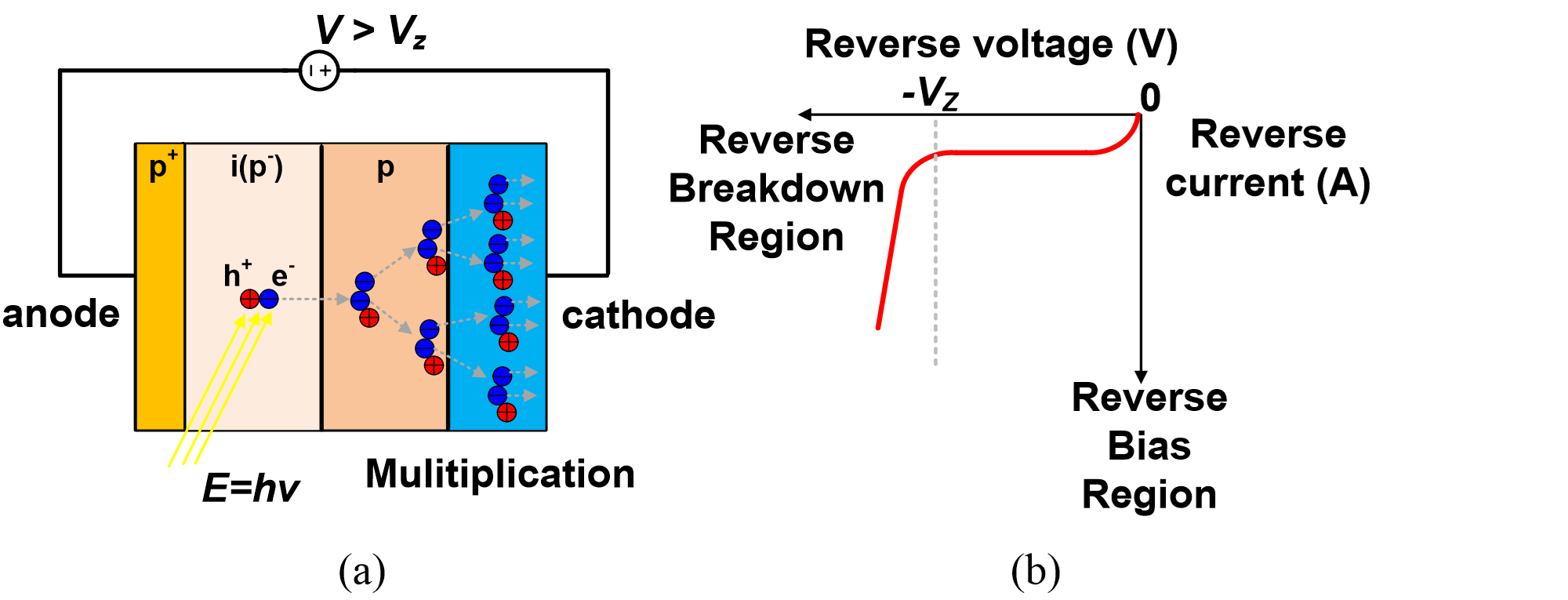## II. ARCHITECTURE

The overall structure of the receiver front-end is shown in Fig. 2. First, the current from the APD passes through the transimpedance amplifier (TIA). Since the signal of TIA is transmitted as single-ended, a regulator is employed to suppress the supply noise. Next, the DC offset cancellation circuit (DCOC) automatically subtracts the DC current of the APD so that the TIA operates at the right bias point. Fig. 3 shows that APD flows an enormous current than PD, implying the vulnerability in DC offset. Therefore, it is essential to employ DCOC for the APD application.

Then, the TIA output and its average voltage, produced by the RC low-pass filter, are forwarded to the single-to-differential amplifier (S2D) to cancel out common-mode noise. Next, the output of the S2D goes through a chain of differential amplifiers and an output buffer for external measurement. They are based on the PMOS current-mode logic (CML).

##### Fig. 2. Overall block diagram of the receiver front-end.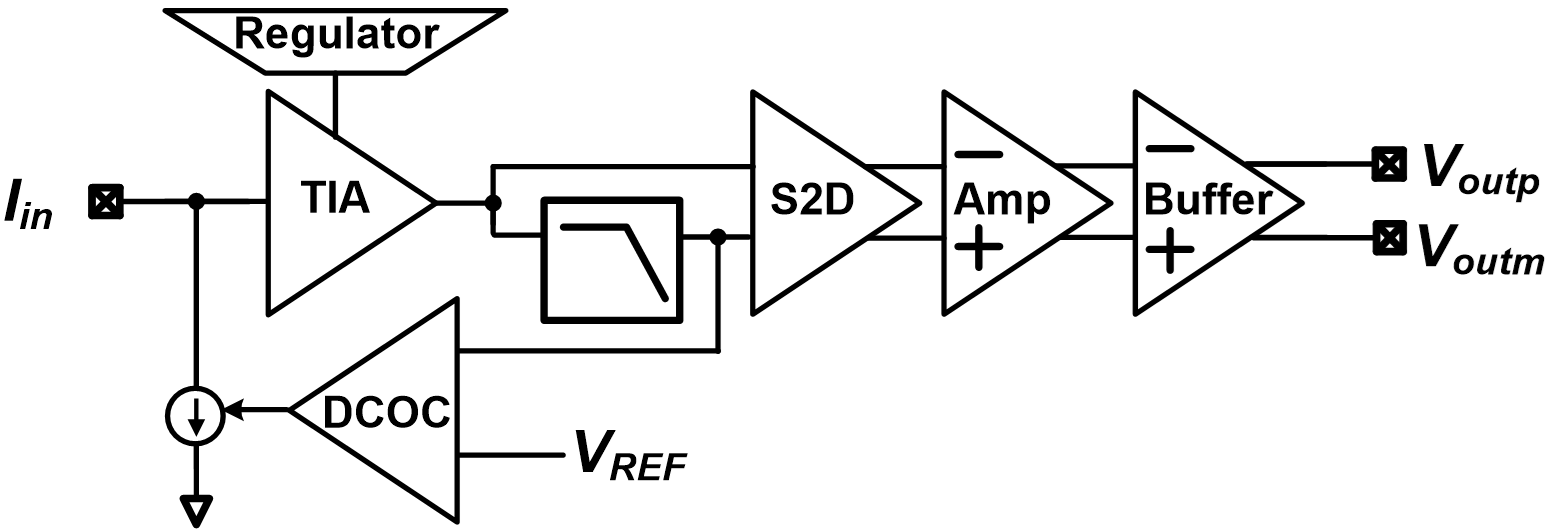##### Fig. 3. The difference of the (a) PD; (b) APD subtraction DC current at the receiver front-end.## III. CIRCUIT DESCRIPTION

### 1. Transimpedance Amplifier (TIA)

The block diagram of TIA is shown in Fig. 4. It consists of a resistive feedback inverter and three cascaded Cherry-Hooper amplifiers (CHAs) to obtain a high gain-bandwidth product (GBW) , and the regulator provides enhanced immunity against supply noise to the TIA. Since a large current flows from the APD, the input DC current effect on the input stage of the TIA is depicted in Fig. 5. The gain of the resistive feedback inverter in response to the input current is described as

##### (2)
$\frac{v_{out}}{i_{in}}=\frac{1-g_{m}R}{g_{m}+\lambda I_{dc}}$

where $g_{m}$ is the transconductance of the inverter, $I_{dc}$ is the DC value of $I_{in}$, ${\lambda}$ is the channel-length modulation parameter, and R is the feedback resistance. Large $I_{dc}$ through the APD reduces the gain of the resistive feedback inverter as calculated by (2). However, with sufficient $g_{m}$, $I_{dc}$ does not significantly affect the gain. Therefore, the inverter is designed to have large $g_{m}$to maintain the same gain even for a large input current.

##### Fig. 5. Small-signal analysis at input stage of TIA.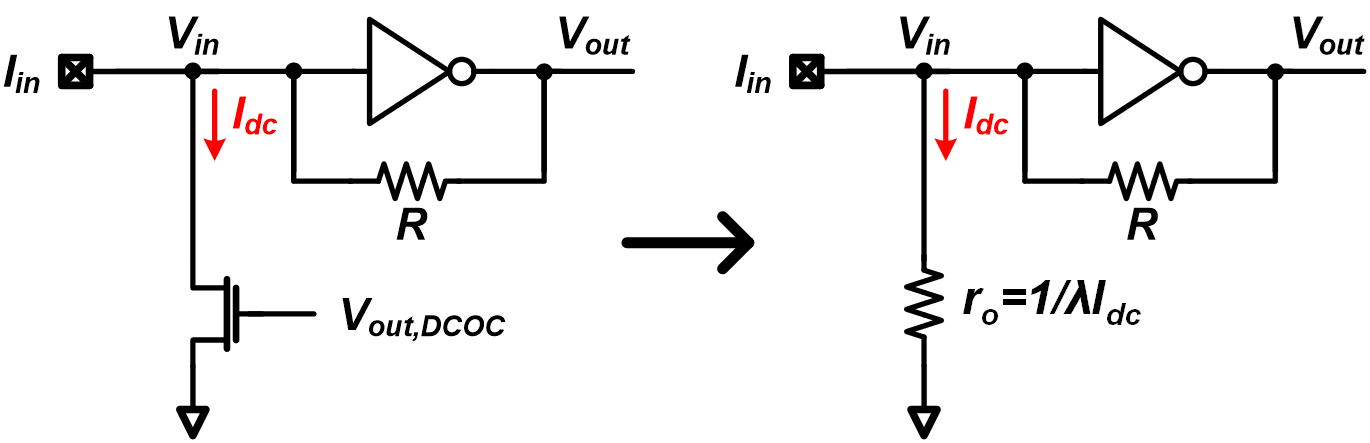### 2. Inverter-based Cherry-Hooper Amplifier (CHA)

Fig. 6 shows a small-signal analysis of the CHA, assumed that the sizes of the two inverters are the same. The small-signal gain for Fig. 6 is written by

##### (3)
$\left| \frac{v_{out}}{v_{in}}\left(iw\right)\right| =\frac{g_{m}R_{F}-1}{\left| \left(1-\frac{w^{2}R_{F}{C_{inv}}^{2}}{g_{m}}\right)+i\left(\frac{2wC_{inv}}{g_{m}}\right)\right| }$

where $C_{inv}$ is load capacitance and $g_{m}$ is the transconductance of the inverters and $R_{F}$ is the feedback resistor of the CHA. The 3 dB bandwidth condition, which means the denominator is $\sqrt{2}$, can be derived as

##### (4)
$w_{-3dB,CHA} =\frac{\sqrt{g_{m}R_{F}-2+\sqrt{\left(g_{m}R_{F}-2\right)^{2}+\left(g_{m}R_{F}\right)^{2}}}}{R_{F}C_{inv}}\\ =\frac{N}{R_{F}C_{inv}}.$

Thus, the equation representing GBW in Fig. 6 can be expressed as follows:

##### Fig. 6. Small signal analysis of CHA.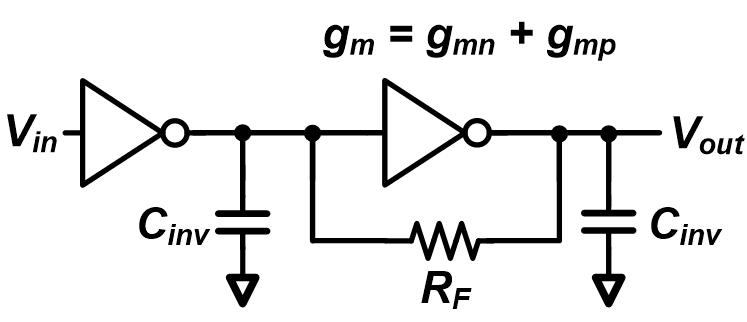##### (5)
$\mathrm{GBW}_{\mathrm{CHA}}=\frac{\left(g_{m}R_{F}-1\right)N}{R_{F}C_{inv}}.$

$g_{m}$ and $C_{inv}$increase proportionally as the width of the transistor increases and the value of N which is the numerator of $w_{-3dB,CHA}$ increases as the $g_{m}$$R_{F}value increases. Thus, if the value of g_{m}$$R_{F}$ increases, DC gain increases proportionally, whereas $N$ prevents the bandwidth from decreasing as much as DC gain increases, so the whole GBW increases. As the $g_{m}$$R_{F} value of the CHA increases, the GBW is higher, so it is better to choose a large g_{m}$$R_{F}$value. If you increase the GBW of the CHA by increasing the $g_{m}$ value, the power consumption of the CHA increases, so increase the $R_{F}$value that does not drop the 3 dB bandwidth too much.

### 3. Cherry-Hooper Amplifier (CHA) with Inductor

An inductor is used to extend the bandwidth of the CHA. The CHA shown in Fig. 7 can be analyzed as a shunt feedback inverter driven by the current source. The transfer function is expressed as

##### (6)
$\frac{v_{out}}{i_{in}}=\frac{\left(1-g_{m}R_{F}\right)\left(1+s\frac{g_{m}L}{g_{m}R_{F}-1}\right)}{g_{m}+s\left(C_{out}+C_{in}\right)+s^{2}\left(R_{F}C_{out}\right)+s^{3}LC_{out}}$

where the input and output parasitic capacitances are $C_{in}$ and $C_{out}$, respectively and $g_{m}$is the transconductance of the inverter. The output resistance of the NMOS and PMOS transistors is assumed to be large enough. As the inductor and resistor are connected in series, the impedance increases as the frequency increases. Therefore, the bandwidth is extended.

An inductor can be used to enhance bandwidth in the input stage of the TIA like Fig. 7. However, placing an inductor in the input stage is costly. The location of the dominant pole is on the input of the TIA due to huge capacitance of the photodiode, the bonding pad, and the ESD diodes. Input parasitic capacitance $C_{in}$ and the input resistance $R_{in}$ are estimated as

##### Fig. 7. Small signal analysis of CHA with inductor.##### (7)
$C_{in}>C_{APD}+C_{pad}\simeq 300\,\mathrm{fF}$

and

##### (8)
$R_{in}=\frac{1}{g_{m}}\simeq 40\Omega$

where $C_{APD}$ is about 100 fF, and $C_{pad}$ is pad capacitance of about 200 fF including ESD diodes. Since $g_{m}$of the inverter is about 25 mS, R$_{F}$ in the TIA is about 250 ${\Omega}$, and output resistance of the transistor is assumed large to be large enough; the required inductance to cancel the dominant pole is estimated as}

##### (9)
$L>\frac{R_{in}C_{in}}{R-1/g_{m}}\,\,\simeq \,\,\,2.52nH.$

Since the required inductance is large, it will occupy a large area. Therefore, increasing the bandwidth at the subsequent stage of the CHA is much more efficient since the resistance and parasitic capacitance are relatively small compared to the input stage. The inductance of 3-stage CHAs in TIA is shown in Fig. 8(a). The 3 dB bandwidth of the TIA is extended from 14 GHz to 29.5 GHz by utilizing inductors in Fig. 8(b), and the peaking of Fig. 8(b) is flattened through the cascaded circuit. The simulation environment of Fig. 8(b) is the same in the following paragraph.

##### Fig. 8. CHA with inductor: (a) inductance and resistance; (b) AC response of TIA by inductor.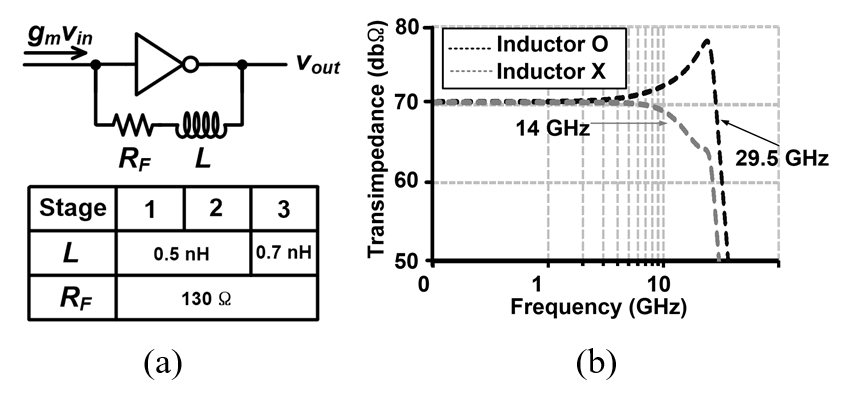## IV. SIMULATION RESULTS

Fig. 9 shows the parasitic elements in measuring the output voltage of the entire receiver system $V_{RX}$. The simulated gain and group delay of $V_{RX}$ are shown in Fig. 10. The pad capacitance $C_{pad}$ is 200 fF, and the printed circuit board (PCB) capacitance $C_{pcb}$ is also 200 fF. The APD capacitance $C_{APD}$ and bond wire inductance $L_{bond}$ is 100 fF and 0.5 nH, respectively. The $C_{ac}$ is AC coupling capacitance. In Fig. 10, the 3 dB bandwidth of the $V_{RX}$is 21.5 GHz, and the maximum difference of group delay is 10 ps below 14 GHz.

##### Fig. 9. The receiver front-end’s parasitic elements modeling.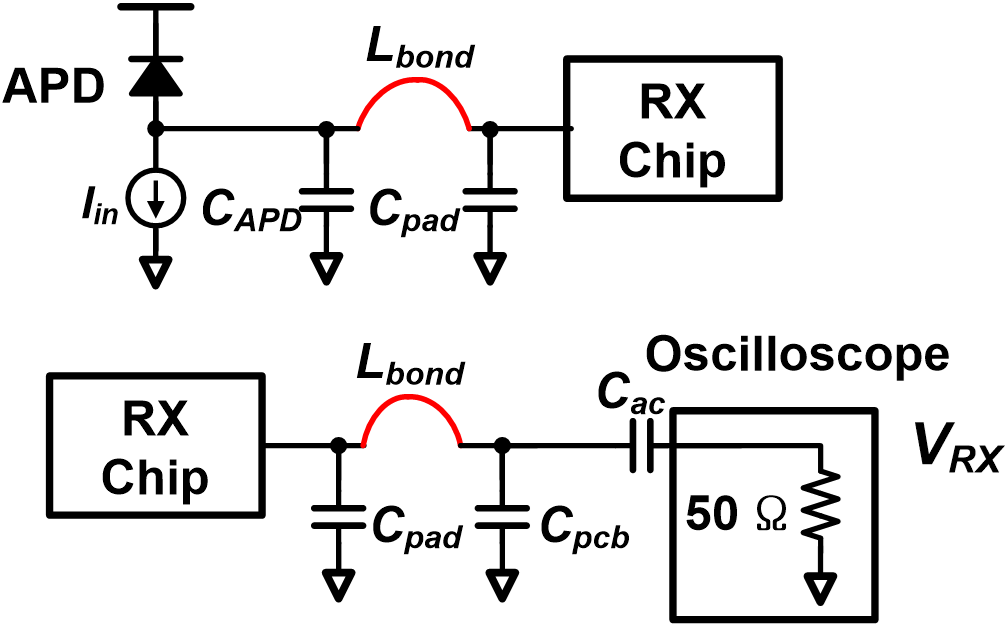##### Fig. 10. The receiver front-end’s gain and group delay. }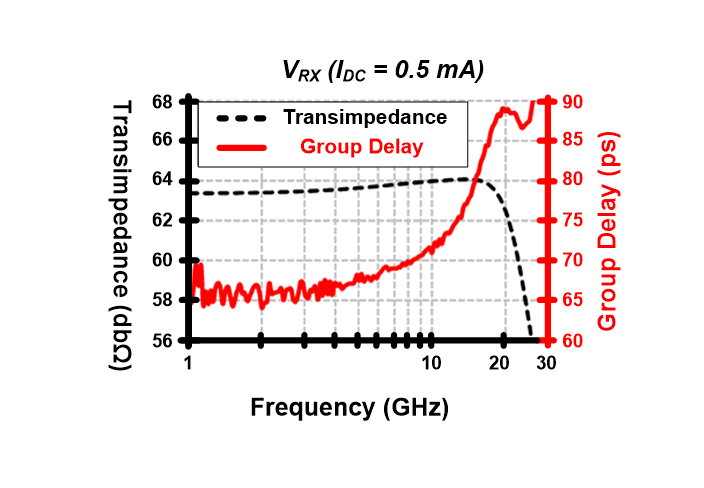## V. MEASUREMENT

The chip is fabricated in a 65 nm CMOS process with the chip photomicrograph shown in Fig. 11(a). The active area of the receiver front-end is 0.22 mm$^{2}$. A large photocurrent of the APD is replaced by equivalent configuration shown in Fig. 11(b). The parallel pattern generator (PPG) transmits a voltage signal in the range of 0 to 3.3 V. C$_{\mathrm{pcb}}$ and L$_{\mathrm{bond}}$ is about 200 fF and 0.5 nH, respectively. C$_{\mathrm{pcb}}$ is comparable for parasitic capacitance of APD. The resistors of 50 ${\Omega}$ and 500 ${\Omega}$ in Fig. 10(b) can transfer up to 6 mA of current to the IC chip. Therefore, the DC value of the input current ranges from 0 to 3 mA. The chip is measured on an FR-4-based PCB. The TIA consumes 12.8 mW, S2D consumes 11.9 mW, and the differential amplifiers consumes 11.8 mW. The output buffer consumes 20.5 mW, but the power of the buffer is excluded since its only purpose is to test performance. Therefore, the entire RX consumes 36.5~mW. The energy efficiency is 1.3 pJ/bit at 28 Gb/s.

Fig. 12 shows the eye diagrams. The eye diagrams are shown for the PRBS 15 pattern when input DC currents are 0.5 mA, 1 mA, 2 mA, and 3 mA, respectively, at 28~Gb/s. The receiver sensitivity is measured by PPG MP1800A (PPG) and BERT Agilent N4903A (BERT). In Fig. 13, the receiver sensitivity in the absence of APD is 340 uA at the BER of 10$^{-12}$. The receiver front-end is designed for high current inputs, so the receiver's gain is relatively low, resulting in the above results. In Table 1, the performance of the receiver front-end is summarized and compared with the prior works. The proposed architecture achieves the highest data rate, while energy efficiency is comparable to others.

##### Fig. 11. (a) Chip Micrograph; (b) measurement setup.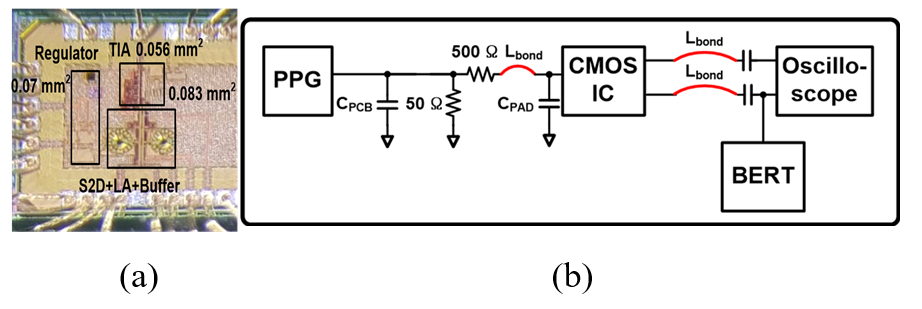##### Fig. 12. Eye diagrams at 28 Gb/s (I$_{dc}$ = (a) 0.5 Ma; (b) 1 mA; (c) 2 mA; (d) 3 mA).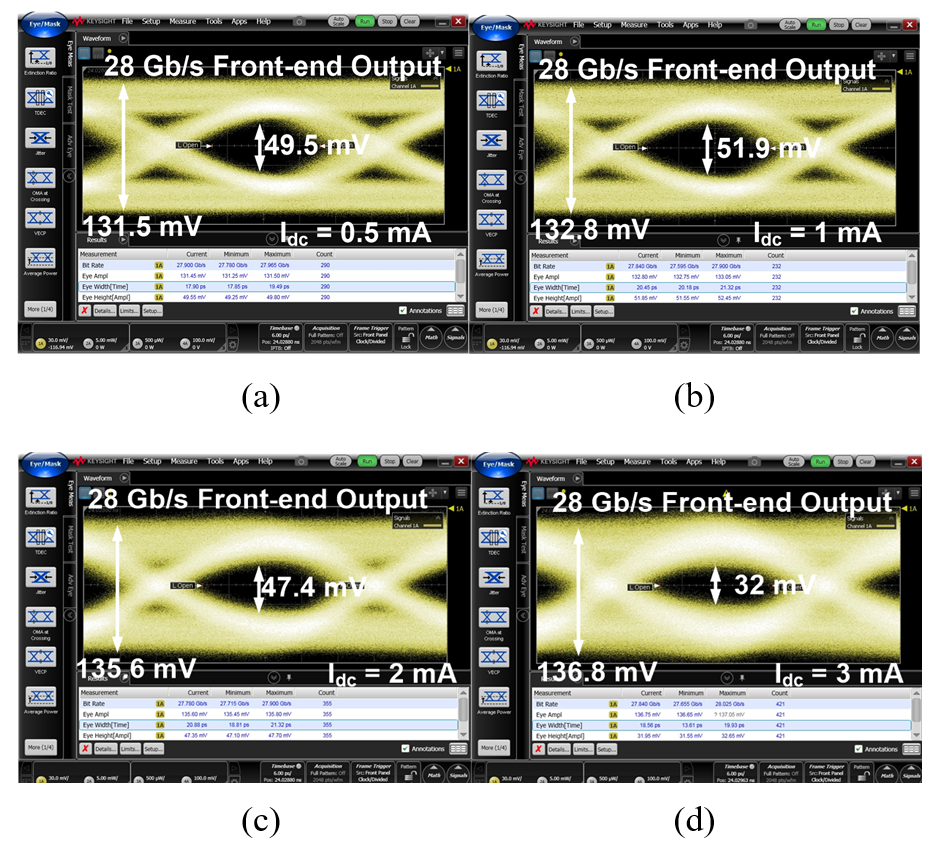##### Fig. 13. Sensitivity of receiver front-end.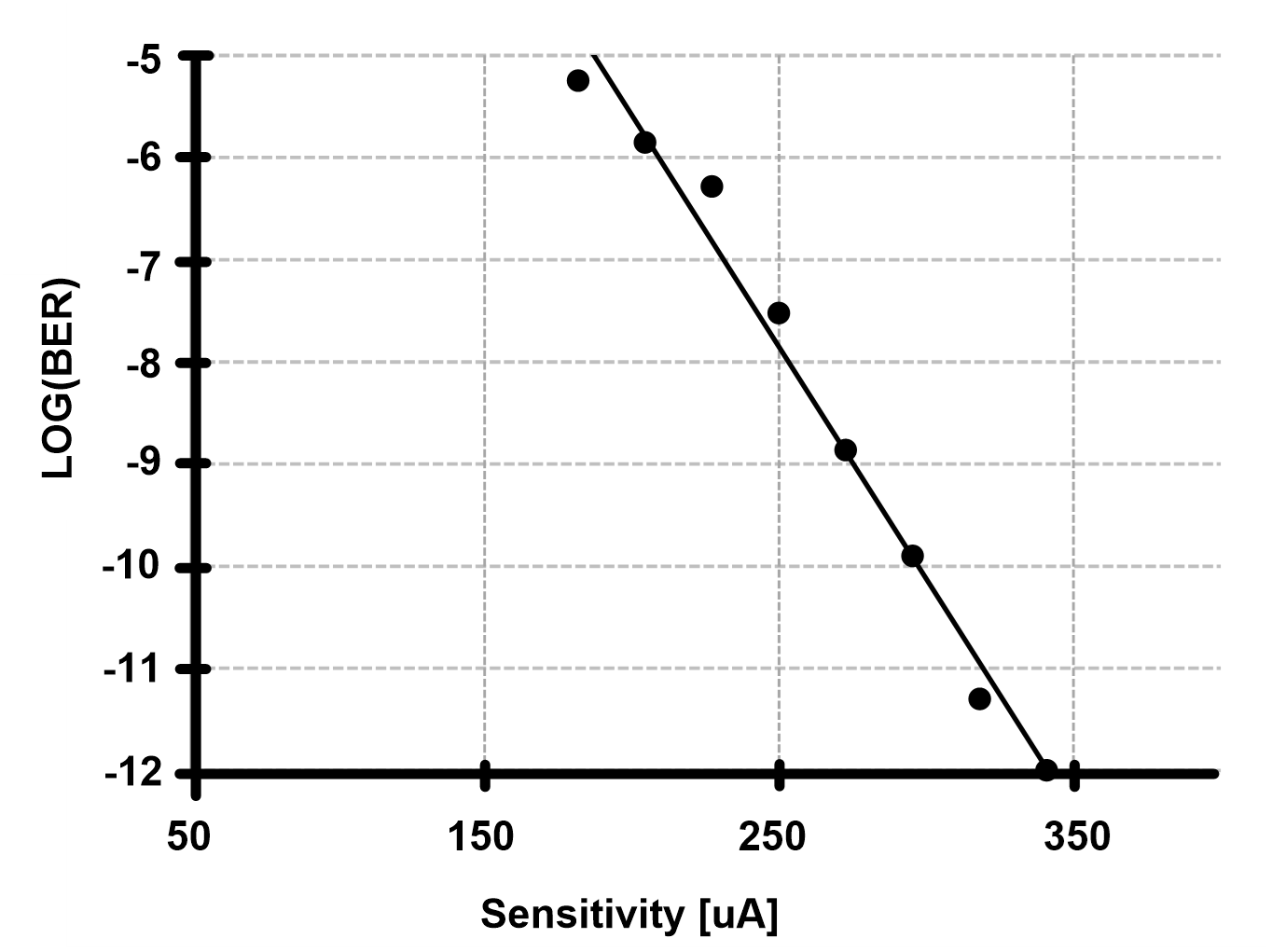##### Table 1. Comparison Table
     This work Technology 65 nm CMOS 40 nm CMOS 65 nm CMOS 65 nm CMOS 65 nm CMOS Data rate (Gb/s) 20 25 18 25-28 28 Energy Efficiency(pJ/bit) 0.71 1.13 2.7 3.25 1.3 Data Type PRBS 7 PRBS 15 PRBS 15 PRBS 31 PRBS 15 Area (mm2) 0.027 0.007 0.025 0.032 0.022 Sensitivity 131 $\mu$A$_{\mathrm{pp}}$ 53 $\mu$A$_{\mathrm{pp}}$ 88 $\mu$A$_{\mathrm{pp}}$ 86 $\mu$A$_{\mathrm{pp}}$ 340 $\mu$A$_{\mathrm{pp}}$

## VI. CONCLUSIONS

The receiver front-end to amplify the photocurrent of the APD is designed in a 65 nm CMOS process. The TIA automatically subtracts any high DC input typically exhibited in the high-gain APD and maintains a robust gain. In addition, the bandwidth extension techniques are used to achieve high data rates by adding a feedback inductor to the CHA. The operation is confirmed at 28 Gb/s with various DC current levels, where the power consumption and power efficiency are 36.5 mW and 1.3 pJ/bit, respectively.

## ACKNOWLEDGMENTS

This work has been supported by the Electronics and Telecommunications Research Institute (ETRI) and the Institute of Information & Communications Technology Planning & Evaluation (IITP) Grant funded by the Korea Government (MSIT) (Development of Multi-Rate, Ultra-High-Speed Links With 100+Gbps Aggregate Bandwidth for AI Computing Platforms) under Grant 220-0-01307.

## References

1
Nada M., Yoshimatsu T., Muramoto Y., Yokoyama H., Matsuzaki H., 1 March1, 2015, Design and Performance of High-Speed Avalanche Photodiodes for 100-Gb/s Systems and Beyond, in Journal of Lightwave Technology, Vol. 33, No. 5, pp. 984-990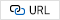2
Zhou Gaolei, 2021, 25 Gbps inductorless optical receiver analog front-end based the modified Cherry-Hooper amplifier for optical interconnect, in Microelectronics Journal, Vol. 1133
Ong D. S. G., Hayat M. M., David J. P. R., Ng J. S., Feb.15, 2011, Sensitivity of High-Speed Lightwave System Receivers Using InAlAs Avalanche Photodiodes, in IEEE Photonics Technology Letters, Vol. 23, No. 4, pp. 233-2354
Sharif-Bakhtiar A., Chan Carusone A., Nov. 2016, A 20 Gb/s CMOS Optical Receiver With Limited-Bandwidth Front End and Local Feedback IIR-DFE, in IEEE Journal of Solid-State Circuits, Vol. 51, No. 11, pp. 2679-26895
Huang S., Chen W., March 2017, A 25 Gb/s 1.13 pJ/b ${-}$10.8 dBm Input Sensitivity Optical Receiver in 40 nm CMOS, in IEEE Journal of Solid-State Circuits, Vol. 52, No. 3, pp. 747-7566
Pan Q., Wang Y., Lu Y., Yue C. P., Nov.-Dec. 2016, An 18-Gb/s Fully Integrated Optical Receiver With Adaptive Cascaded Equalizer, in IEEE Journal of Selected Topics in Quantum Electronics, Vol. 22, No. 6, pp. 361-3697
Takemoto T., Yamashita H., Yazaki T., Chujo N., Lee Y., Matsuoka Y., Oct. 2014, A 25-to-28 Gb/s High-Sensitivity (${-}$9.7 dBm) 65 nm CMOS Optical Receiver for Board-to-Board Interconnects, in IEEE Journal of Solid-State Circuits, Vol. 49, No. 10, pp. 2259-2276Daehyun Koh received the B.S. degree in electrical engineering from the Seoul National University, Seoul, South Korea, in 2017, where he is currently pursuing the Ph.D. degree. His current research interests include the design of high-speed I/O circuits, optical interface circuits, and memory systems.

Gyu-Seob Jeong (Daniel Jeong) received the B.S. and Ph.D. degrees in electrical engineering and computer science from Seoul National University, Seoul, South Korea, in 2012 and 2017, respectively. From 2017 to 2019, he was a Postdoctoral Researcher with Seoul National University. In 2019, he joined Ayar Labs, where he has been working as a Circuit Design Engineer. His research interests include optical interconnects and high-speed I/O circuits.

Jeongho (Jeffrey) Hwang received the B.S. and Ph.D. degrees in electrical engineering and computer science from Seoul National University, Seoul, South Korea, in 2014 and 2019, respectively. In 2019, he was a Postdoctoral Researcher with the Inter-University Semiconductor Research Center, Seoul National University. He joined Cadence Design Systems, in 2020, where he is currently a Principal Design Engineer. His research interests include high-speed I/O interfaces, silicon photonics, and on-chip reference oscillators. Dr. Hwang was a recipient of Korea Foundation for Advanced Studies during 2011–2018, IEEE CASS Student Travel Grant Award in 2019, IEEE SSCS Student Travel Grant Award in 2019, Cadence Design Systems Rising Star Award in 2022.

Deog-Kyoon Jeong received the B.S. and M.S. degrees in electronics engineering from Seoul National University, Seoul, South Korea, in 1981 and 1984, respectively, and the Ph.D. degree in electrical engi-neering and computer sciences from the University of California at Berkeley, Berkeley, CA, USA, in 1989. From 1989 to 1991, he was with Texas Instruments, Dallas, TX, USA, as a Member of the Technical Staff and worked on the modeling and design of Bipolar CMOS (BiCMOS) gates and the single-chip implementation of the Scalable Processor ARChitecture (SPARC). He joined the Faculty of the Department of Electronics Engineering and InterUniversity Semiconductor Research Center, Seoul National University, where he is currently a Professor. He was one of the cofounders of Silicon Image (now Lattice Semiconductor), Sunnyvale, CA, USA, which specialized in digital interface circuits for video displays, such as digital visual interface (DVI) and high definition multimedia interface (HDMI). His main research interests include the design of high-speed I/O circuits, phase-locked loops (PLLs), and memory system architecture.